# Depletion-Based Stock Stock Reduction Analysis (DB-SRA)

Depletion-Based Stock Reduction Analysis (DB-SRA) is an assessment method for data-limited fisheries in cases where approximate catches are known from the start of exploitation, but there are no indices of abundance or informative composition data. DB-SRA requires an approximate natural mortality rate (M) and age at maturity. A production function is specified based on the relative location of maximum productivity to carrying capacity, and the relationship of FMSY to M. Given a probability distribution for the depletion level (i.e. B/B0) near the end of the time series, the method derives a probability distribution for unfished biomass B0, and distributions for management reference points. The method was published by Dick and MacCall (Fisheries Research 110:331-341). Arnold and Heppell have evaluated DB-SRA using retrospective analysis.

DB-SRA is best suited for situations in which there is a time-series of historical catches, ideally from the start of the fishery. Also, DB-SRA requires priors on multiple parameters. Some of these can be inferred from the literature but the prior on recent depletion (biomass relative to carrying capacity) drives the results to a substantial extent. Senstivity to this prior should be ideally be examined.

# Installation

This package is run from R and installed from CRAN. R can be installed from https://cran.r-project.org/.

The recommended way to run R is using RStudio. After you have installed R, then install RStudio. It can be found at https://www.rstudio.com/products/rstudio/download/.

There are several implementations of DB-SRA. The supported version of DB-SRA is available from the fishmethods package within R. Another version can be found in the Data-Limited Methods Toolkit. To use DB-SRA, you first need to install the fishmethods package.

A reference manual for fishmethods and therefore dbsra can be found at “https://cran.r-project.org/web/packages/fishmethods/fishmethods.pdf”.

If you are using this code for the first time, then you need to change eval=FALSE to eval=TRUE.

``````Check_Install.packages <- function(pkg){
new.pkg <- pkg[!(pkg %in% installed.packages()[, "Package"])]
if (length(new.pkg))
install.packages(new.pkg,dependencies = TRUE) else print(paste0("'",pkg,"' has been installed already!"))
sapply(pkg, require, character.only = TRUE)
}

Check_Install.packages("fishmethods")``````
``##  "'fishmethods' has been installed already!"``
``## Loading required package: fishmethods``
``## Warning: package 'fishmethods' was built under R version 4.0.3``
``````## fishmethods
##        TRUE``````

NB Once you have installed fishmethods, you don’t need to run the install.packages code chunk again.

``library(fishmethods)``

``````Example <- read.csv("Catches.csv")
# Read in the simulated annual catch data set
Example <- Example[-c(1:11),c(1,3)]
# We have removed the first 11 rows of data as these are all prefishery and zero, also removed the fleet column as only one fleet so not needed
names(Example) <- c("year","catch")
# this makes sure the headings are correct for later use
``````##    year     catch
## 12 1971   98.9982
## 13 1972  199.1610
## 14 1973  991.5380
## 15 1974  988.8430
## 16 1975 1978.3500
## 17 1976 1999.4000``````

Our data set was created using Stock Synthesis based on an age at 50% maturity of roughly 10-11 years and a rate of natural mortality of 0.2yr-1 for females and 0.25yr-1 for males. The catch data are in metric tons.

## Description of the model settings

DB-SRA is run using the function dbsra. There are many inputs to this function. These can be divided into general (required) inputs, those related to the priors and those related to the other aspects of the assessment.

• general (required) inputs:
• year: a vector of the years for which historical catches are available.
• catch: the vector of the historical catches corresponding to the vector year.
• catchCV: a vector of CVs to capture uncertainty in catches (catchCV=NULL implies that catches are known exactly and therefore catchCV is not required).
• priors and constraints:
• catargs: specifies how catch uncertainty is quantified using a list list(dist,low,up,unit). The options for the probability distribution for the historical catches are: norm for normal catches, lnorm for log-normally catches, and unif for uniformally distributed catches. The unit argument specifies the unit for reporting. For example catargs=list(dist=“unif”,low=catch0.9,up=catch1.1,unit=“MT”) would specify that the historical catches are uniform between 90% and 110% of the values specified in catch argument.
• maxn: (default 25) the model computes the Pella-Tomlinson shape parameter and this parameter places an upper bound on it.
• k: specifies the bounds on carrying capacity using a list list(low,up,tol,permax). The computed carrying capacities are constrained to be between the up and low arguments. Only simulations that match the generated and computed final depletion within the tol argument (default 0.01) are included in the final sample.
• b1k: specifies the prior for the relative depletion level (B1K) in the first year using a list list(dist,low,up,mean,sd). The options for the selected distribution dist argument are: (a) none (B1K equals the mean), (b) unif (B1K is drawn between the low and up parameters), (c) norm, lnorm, gamma, and beta (the distribution for B1K is defined by the parameters mean and sd within bounds defined by low and up.
• btk: specifies the prior for the relative depletion level in a reference year using a list list(dist,low,up,mean,sd,refyr). The parameters are specified as for b1k.
• M: specifies the prior for the rate for natural mortality (M) using a list list(dist,low,up,mean,sd). The parameters are specified as for b1k.
• fmsym: specifies the prior for the rate for FMSY/M using a list list(dist,low,up,mean,sd). The parameters are specified as for b1k.
• bmsyK: specifies the prior for the rate for BMSY/K using a list list(dist,low,up,mean,sd). The parameters are specified as for b1k.
• other inputs
• agemat: specifies the age (in years) that animals enter into the reproductive biomass.
• nsims: number of Monte Carlo samples. This number should ideally be 10,000 or larger.
• grout: send the output the console (1) or to both console and a TIF files (2). The file names are automatically generated if a TIF file option is selected.
• graphs: the graphs that can be produced depend on this argument:
• 1: line plot of observed catch versus year,
• 2: histogram of K values
• 3: histogram of BMSY values
• 4: histogram of MSY values
• 5: histogram of FMSY values,
• 6: histogram of UMSY (exploitation rate at MSY) values,
• 7: histogram of overfishing limit (OFL) in last year+1 values
• 8: histogram of M values
• 9: histogram of Bt/K values
• 10: histogram of FMSY/M values
• 11: histogram of BMSY/K values
• 12: histogram in biomass in the reference year
• 13: line plots of accepted and rejected biomass trajectories with median and 2.5th and 97.5th percentiles (in red).
• 14: stacked histograms of accepted and rejected values for each input parameter and resulting estimates

## Running the model

The model is run by calling dbsra. The results are the posteriors for the parameters (K, BMSY, MSY, FMSY, UMSY, OFL, BMSY, Bt/K, FMSY/M, BMSY/K, biomass in the last year). The OFL is the catch for the first projection year based on applying FMSY to current biomass. Note that the examples here are based on 100 simulations. This is insufficient for an actual assessment.

The biomass estimates from each simulation are not stored in memory but are automatically saved to a .csv file named “Biotraj-dbsra.csv”. Yearly values for each simulation are stored across columns. The first column holds the likelihood values for each simulation (1 = accepted, 0 = rejected). The number of rows equals the number of simulations (nsims). Thus an error “None of the runs had a likelihood equal to 1” means that none of the runs were accepted.

There are 14 graphs. The default is to produce all of them. A combination of graphs can be selected using the c() statement e.g. c(1,13) or c(1:13).

Below we run the model close to the example settings. This is a reasonably uninformed model (as would often be the case) and shows how DBSRA works in accepting and rejecting various parameter permutations.

``````par(mfrow=c(2,2))
dbsraOut <- dbsra(year = Example\$year,
catch = Example\$catch,
catchCV = NULL,
catargs = list(dist="none",low=0,up=Inf,unit="MT"),
agemat=4,
k = list(low=100,up=500000,tol=0.01,permax=1000),
b1k = list(dist="none",low=0.01,up=0.99,mean=1,sd=0.1),
btk = list(dist="beta",low=0.01,up=0.99,mean=0.3,sd=0.1,
refyr=max(Example\$year)+1),
fmsym = list(dist="lnorm",low=0.1,up=2,mean=-0.223,sd=0.2),
bmsyk = list(dist="beta",low=0.05,up=0.95,mean=0.4,sd=0.05),
M = list(dist="lnorm",low=0.001,up=1,mean=-1.609,sd=0.4),
graph=c(1:14),
nsims = 100,grout=2)``````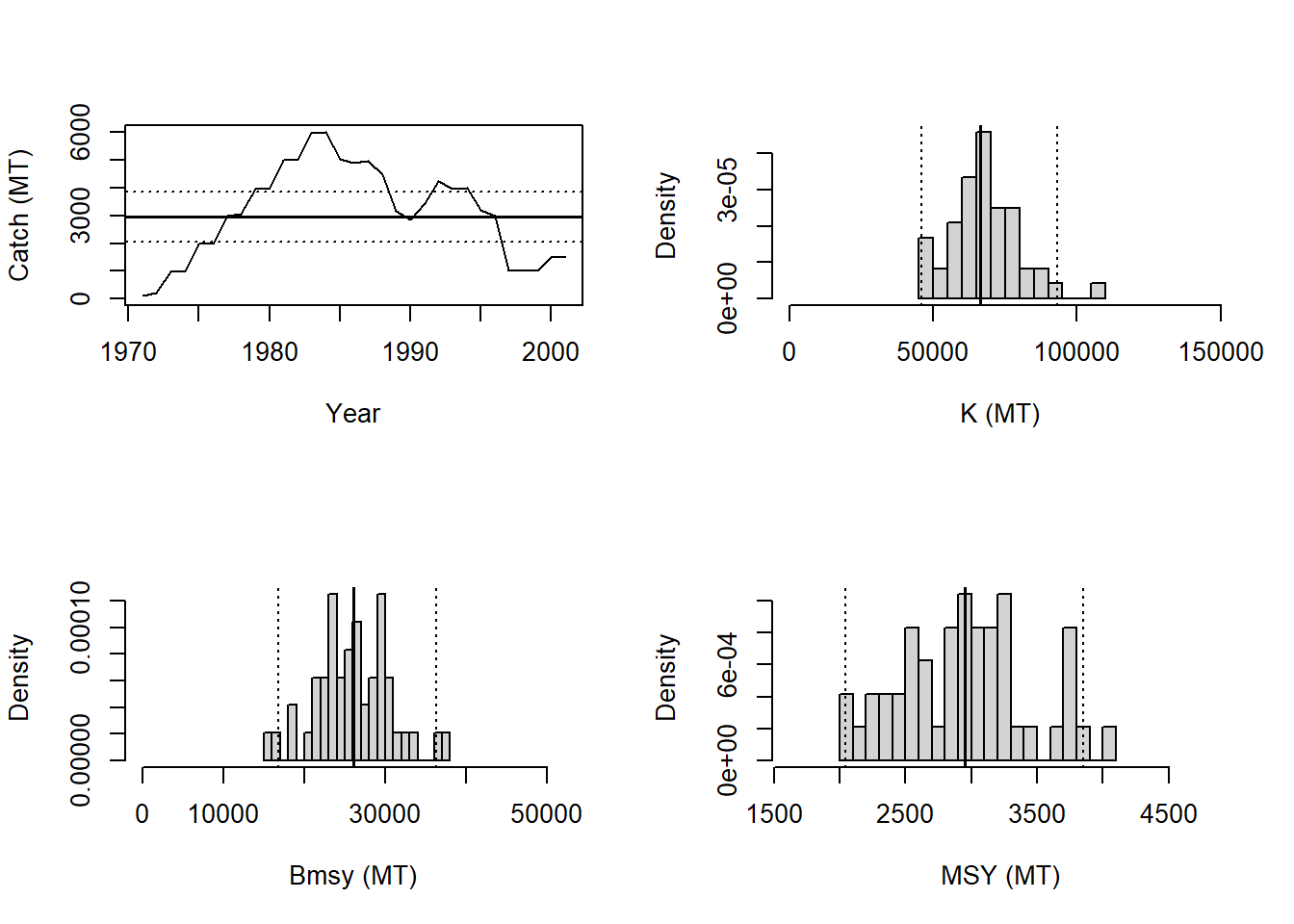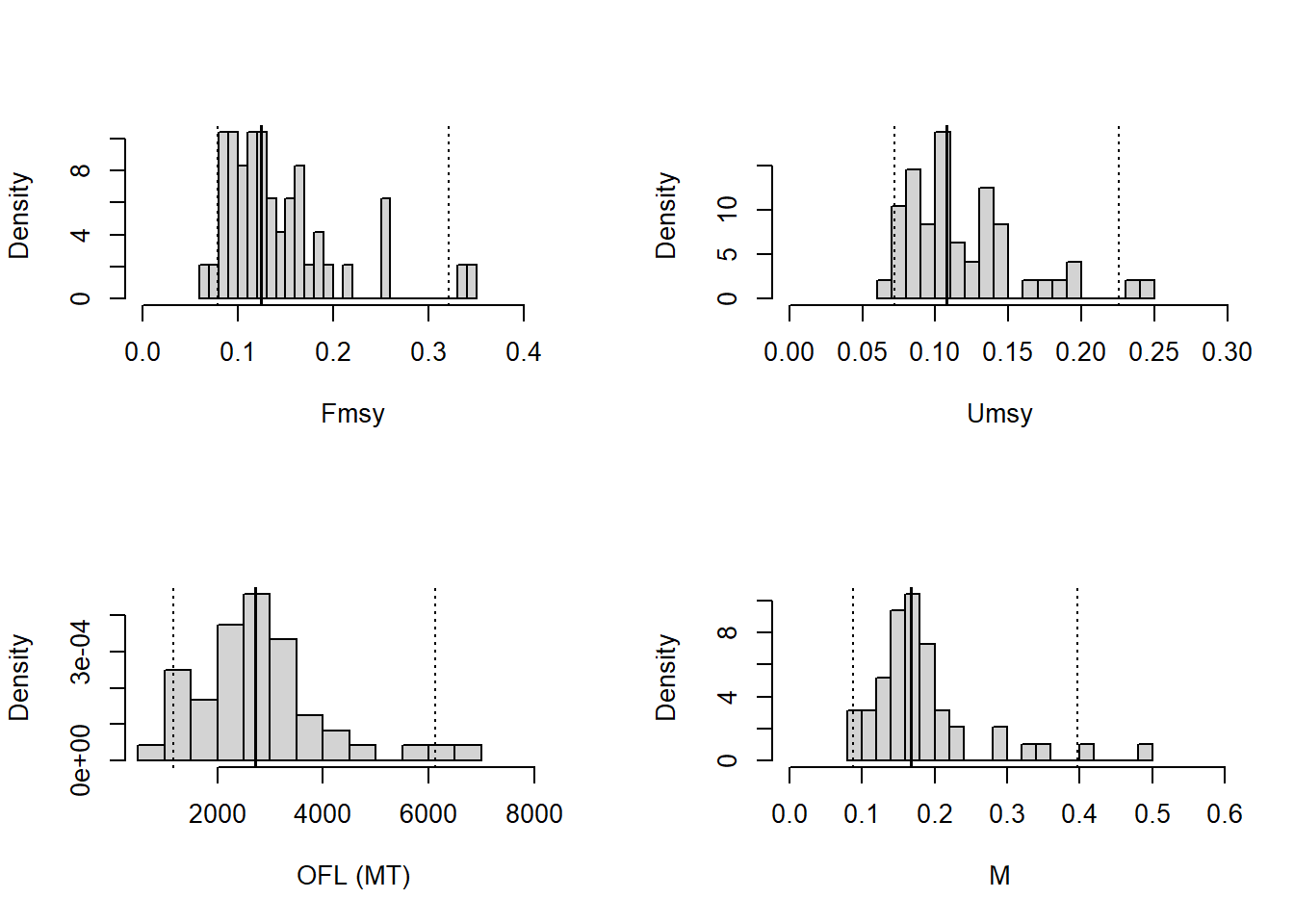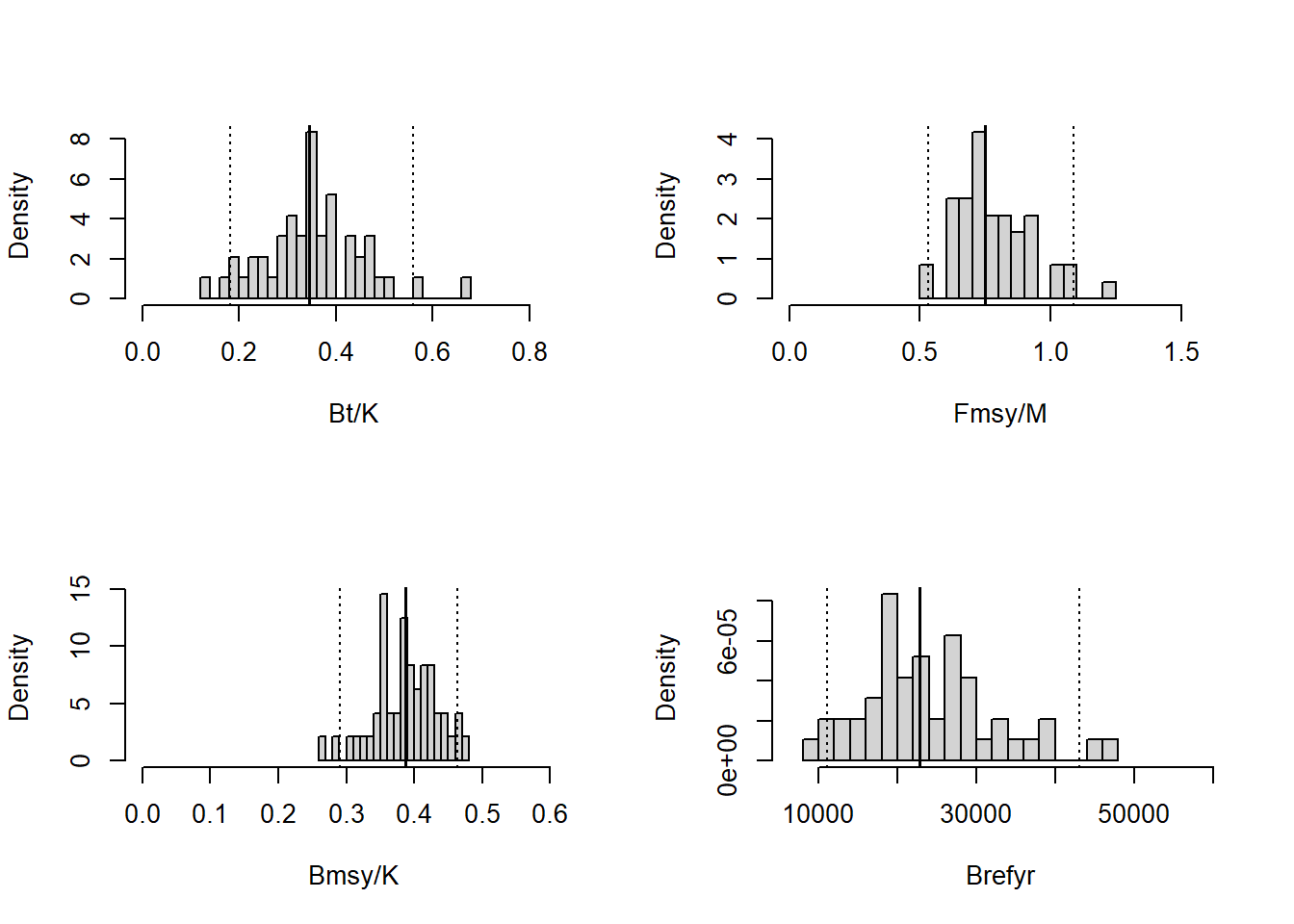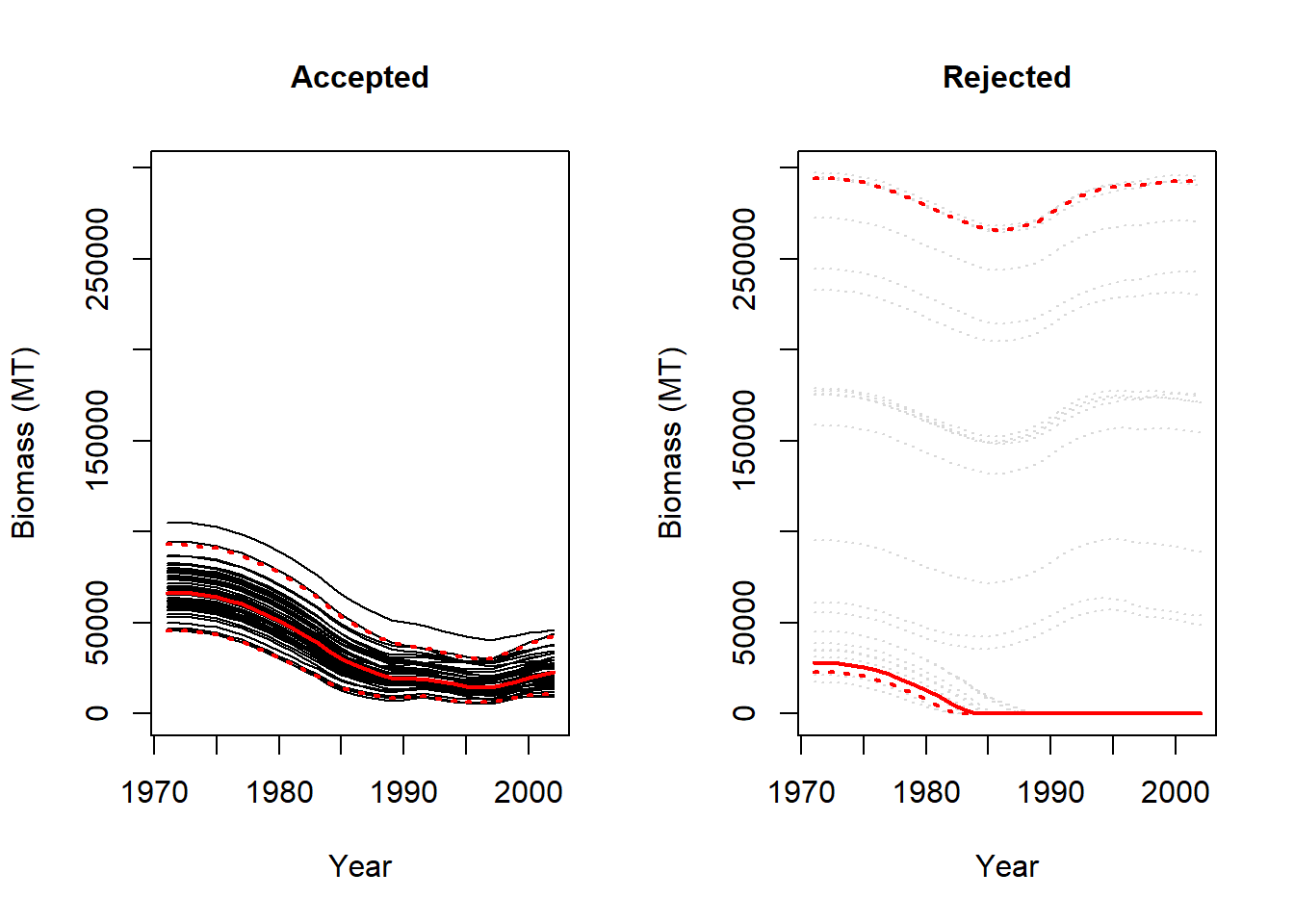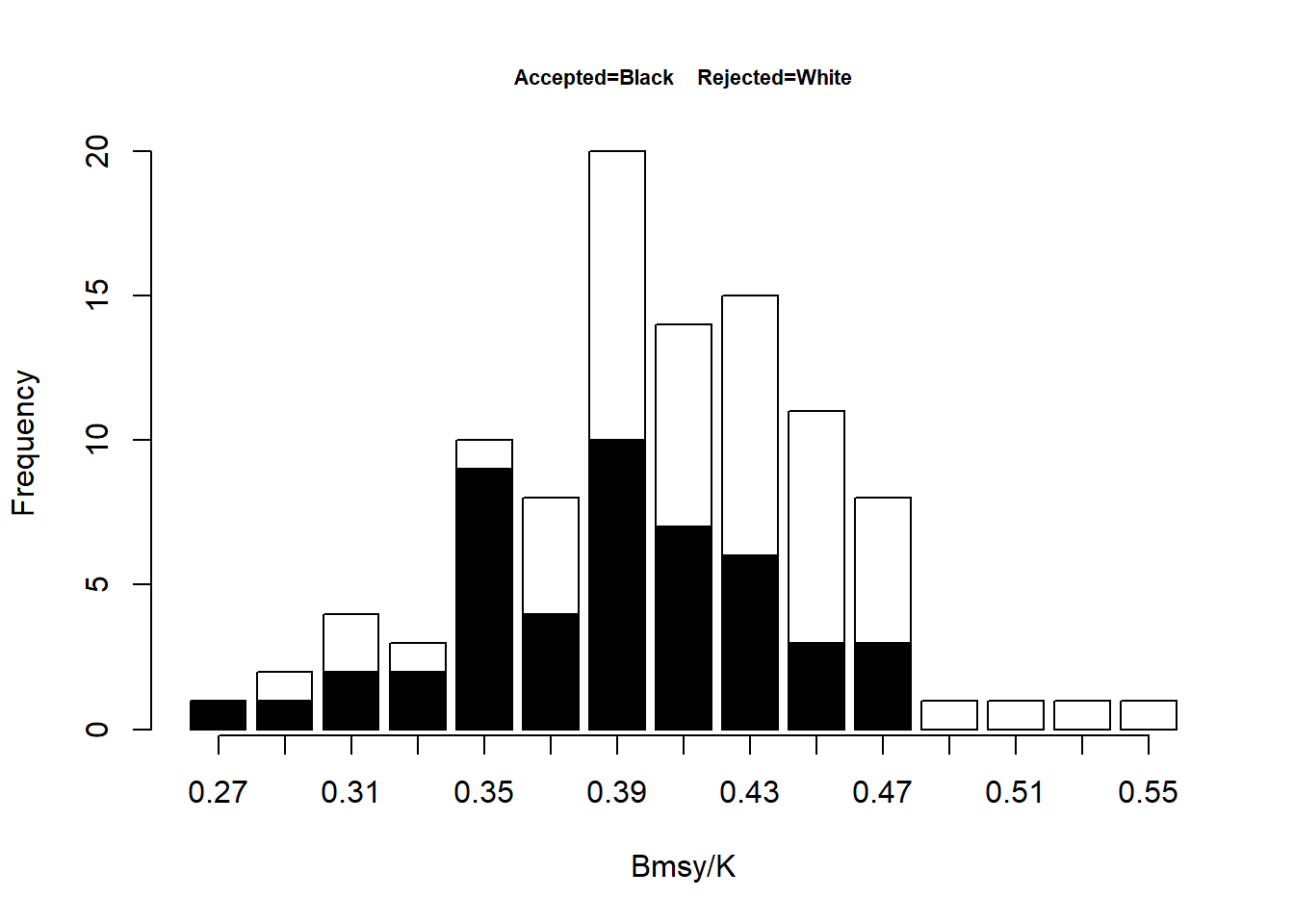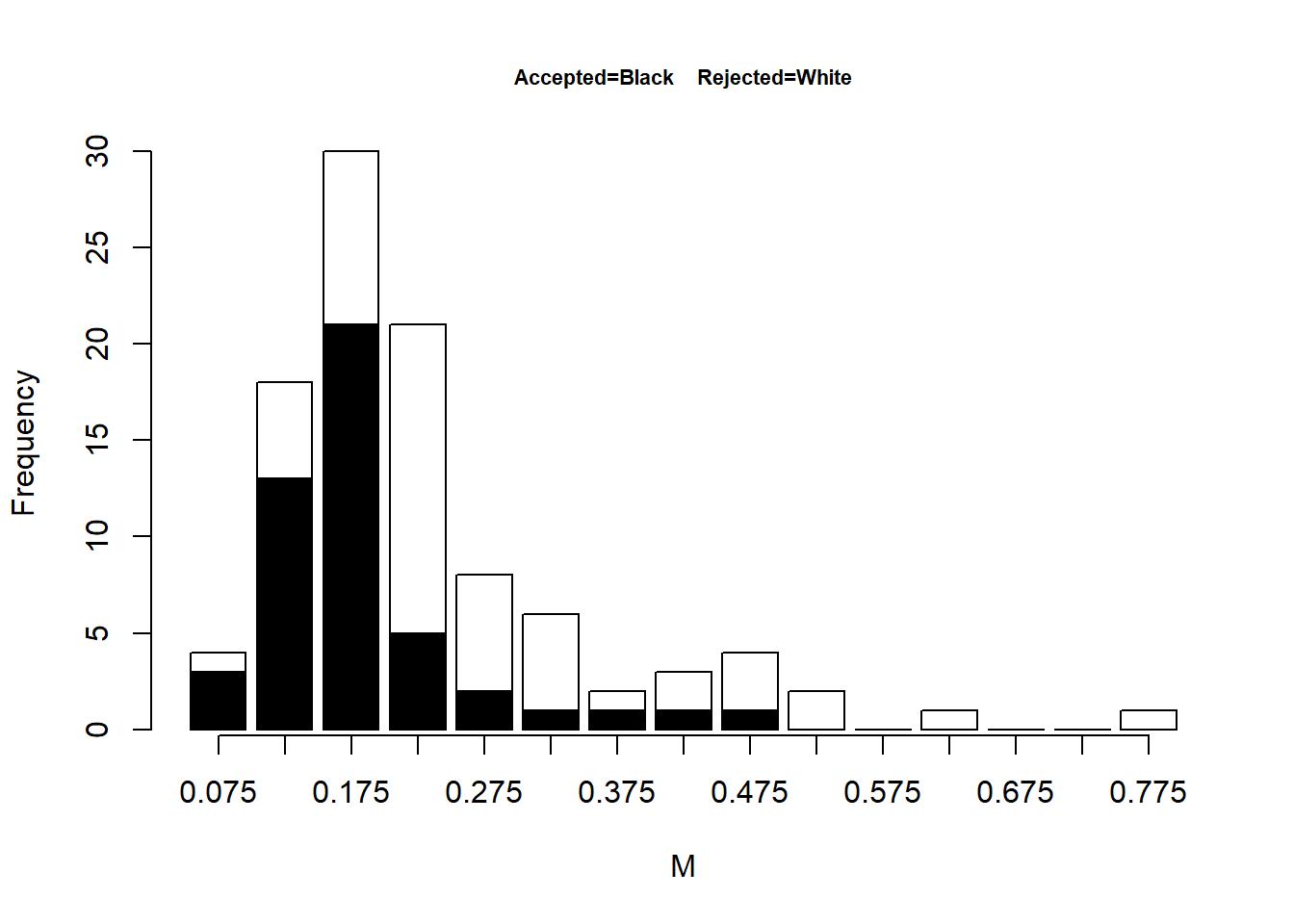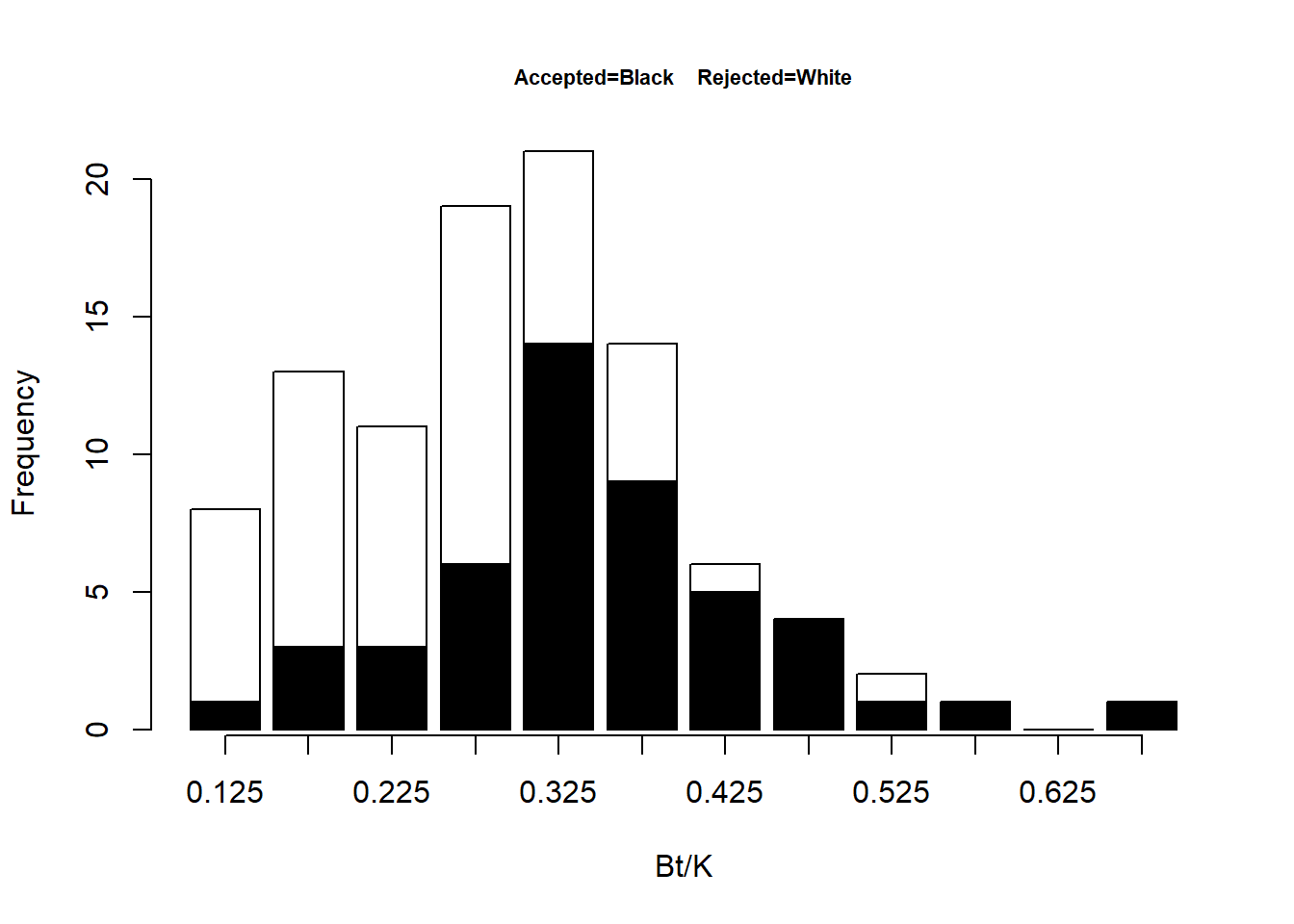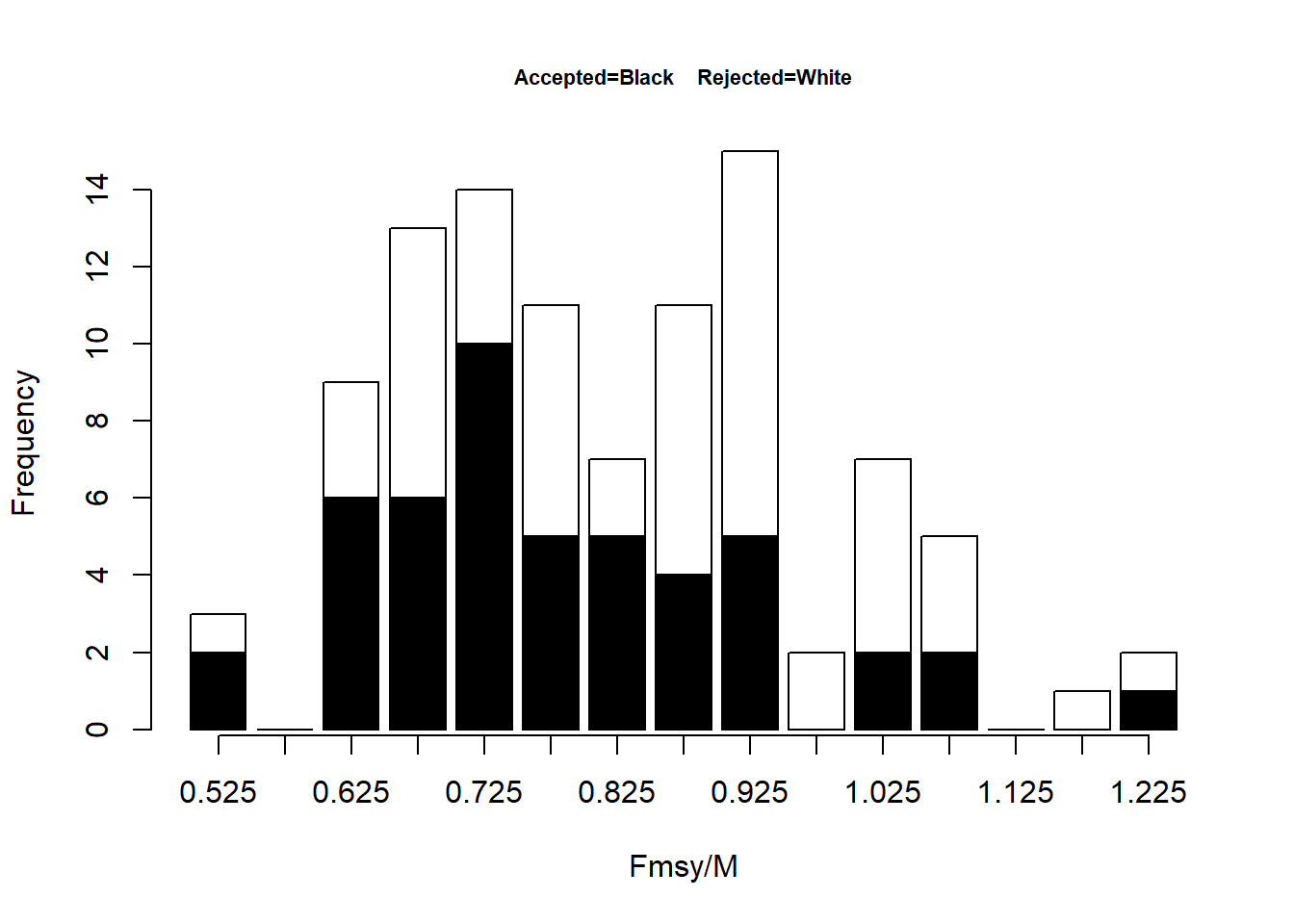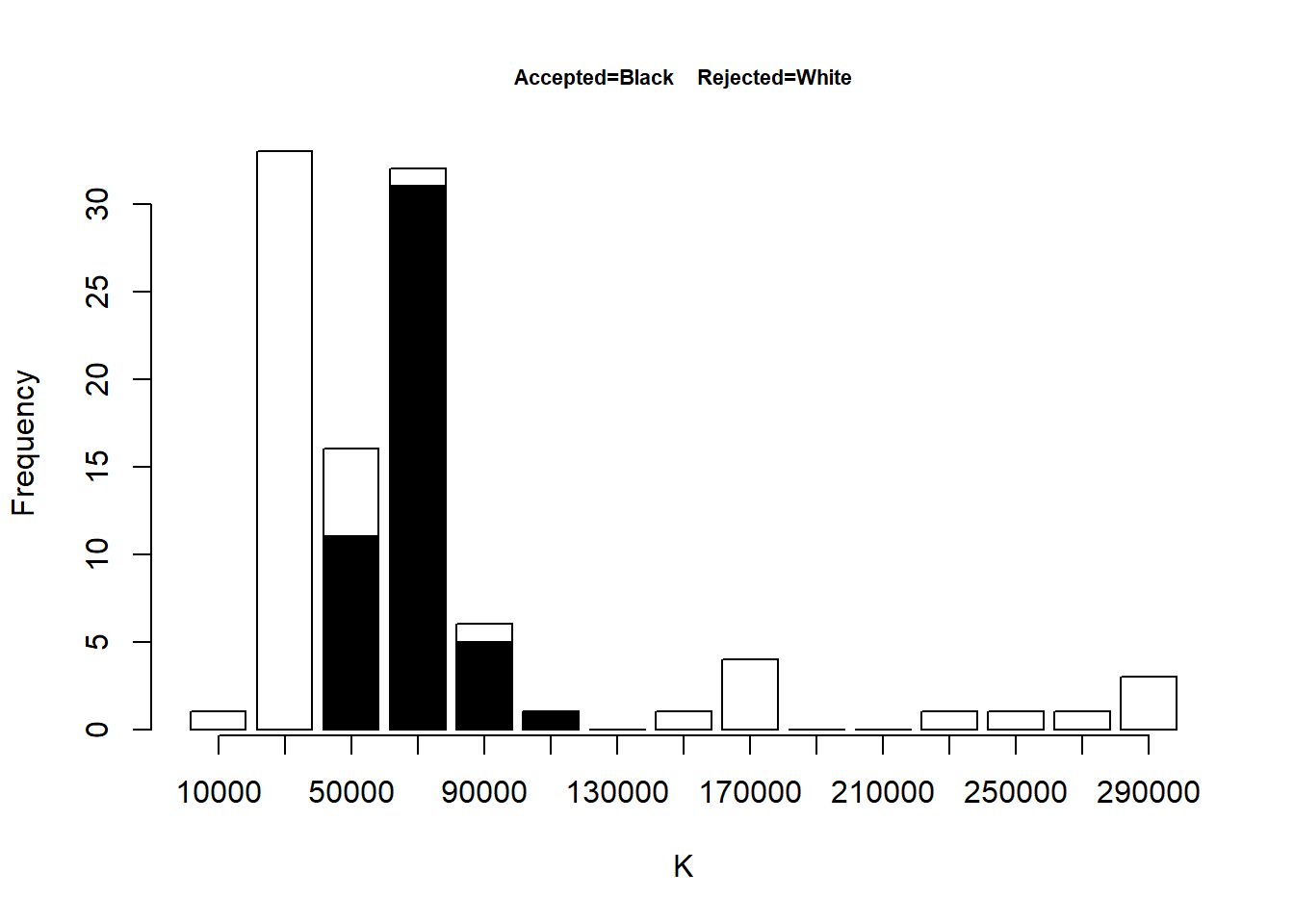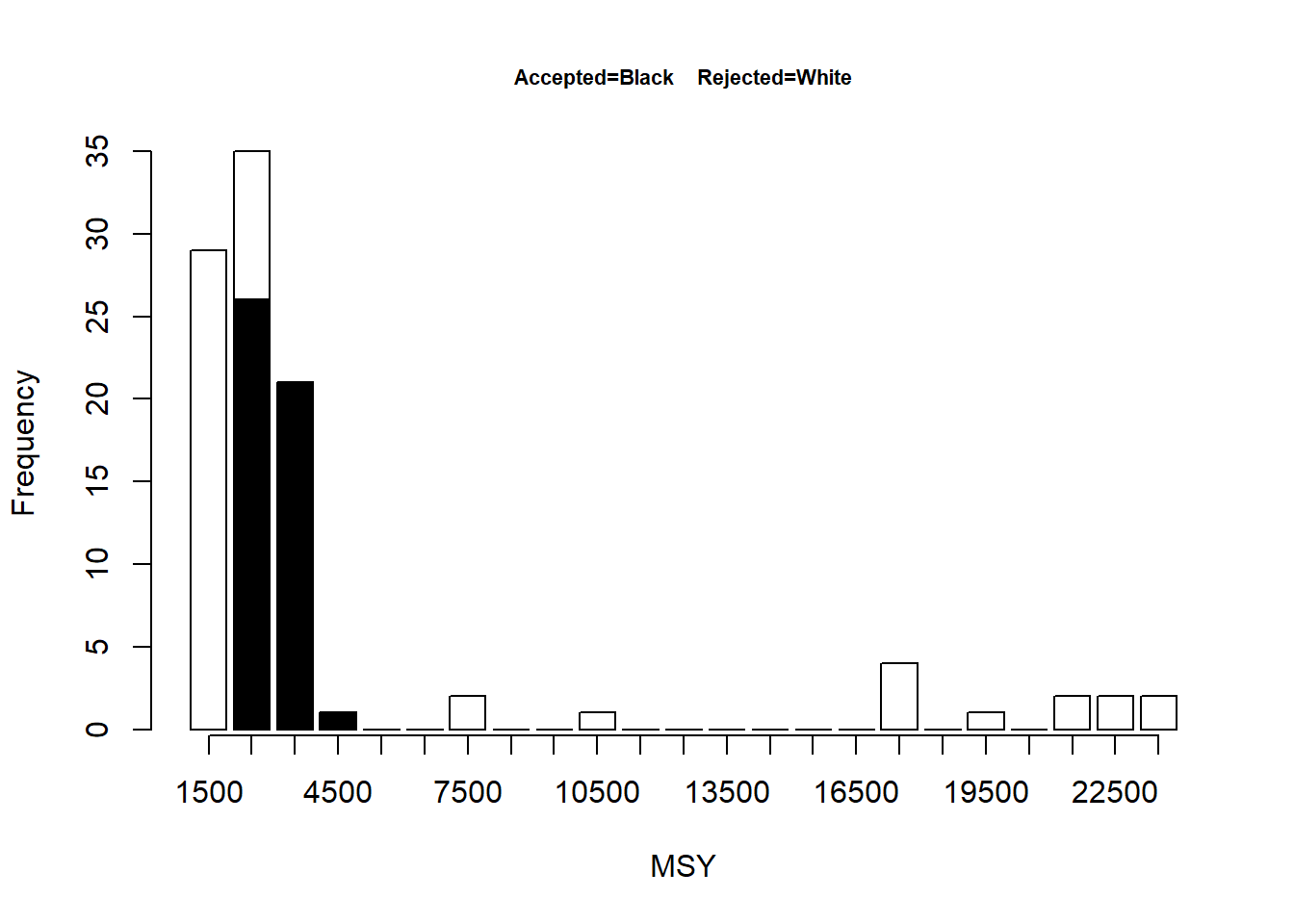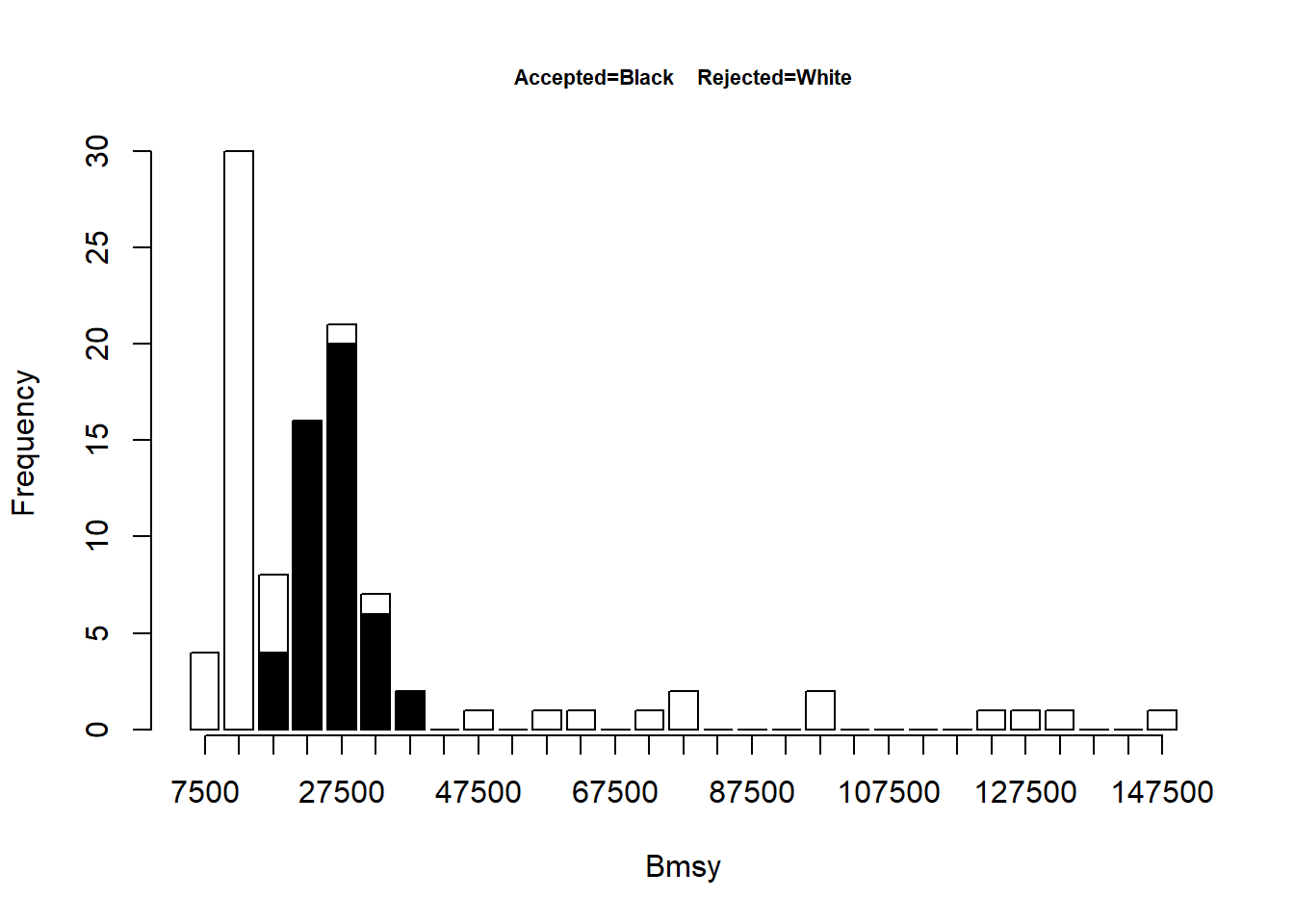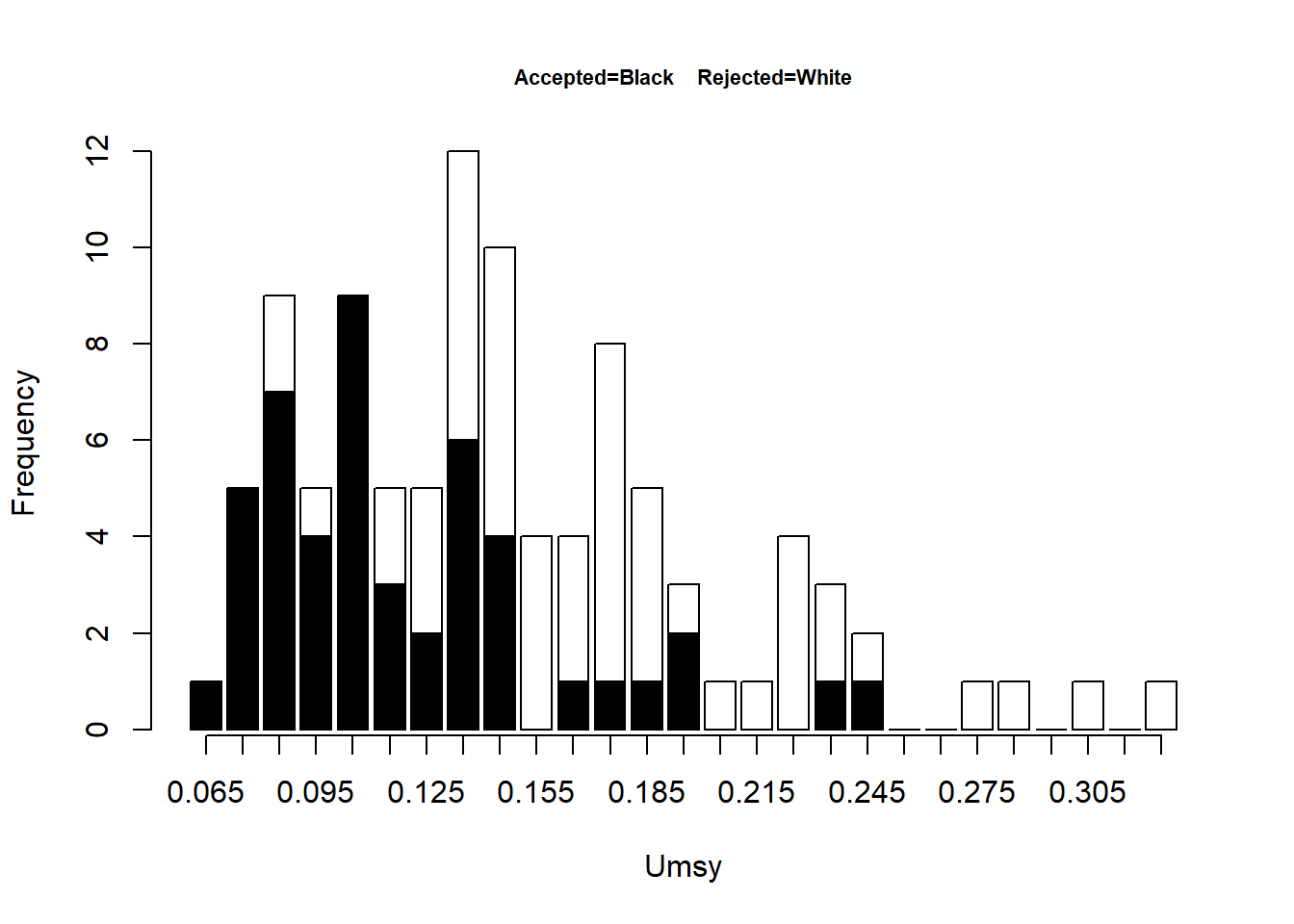The output from the function includes a summary of the specified priors (object Initial), the selected parameters (object Parameters) and the values for the management reference points (object Estimates).

``print(dbsraOut\$Initial)``
``````##        Distr Lower Upper  Mean    SD
## Fmsy/M lnorm   0.1     2 -0.223  0.2
## Br/K    beta  0.01  0.99    0.3  0.1
## Bmsy/K  beta  0.05  0.95    0.4 0.05
## M      lnorm 0.001     1 -1.609  0.4
## refyr   <NA>  2002  <NA>   <NA> <NA>
## B1/K    none  0.01  0.99      1  0.1``````
``print(dbsraOut\$Parameters)``
``````##        Mean (ll=1) Median (ll=1) 2.5% (ll=1) 97.5% (ll=1) min (ll=1) max (ll=1)
## Fmsy/M       0.786        0.7496      0.5304       1.0891 0.51134260  1.2452057
## Bt/K         0.354        0.3459      0.1815       0.5602 0.12823316  0.6728200
## Bmsy/K       0.386        0.3877      0.2901       0.4633 0.26068355  0.4785907
## M            0.185        0.1675      0.0882       0.3971 0.08245986  0.4855176
## B1/K         1.000        1.0000      1.0000       1.0000 1.00000000  1.0000000``````
``print(dbsraOut\$Estimates)``
``````##        Mean (ll=1) Median (ll=1) 2.5% (ll=1) 97.5% (ll=1)   min (ll=1)
## MSY       2954.447     2948.7304   2046.6456    3852.3308 2.022274e+03
## Bmsy     26067.545    26056.3429  16769.7159   36269.9088 1.555801e+04
## Fmsy         0.143        0.1244      0.0793       0.3209 6.744497e-02
## Umsy         0.119        0.1079      0.0722       0.2257 6.248115e-02
## OFL       2816.503     2714.0153   1165.1159    6119.5642 7.248675e+02
## Brefyr   24055.045    22775.4699  11096.4857   43115.6530 9.001708e+03
## K        67944.077    66470.1197  45886.4352   93217.1327 4.564667e+04
##          max (ll=1)
## MSY    4.026953e+03
## Bmsy   3.730537e+04
## Fmsy   3.408840e-01
## Umsy   2.413262e-01
## OFL    6.605754e+03
## Brefyr 4.608397e+04
## K      1.050514e+05``````

The biomass trajectory is saved to a file Biotraj-dbsra.csv in the working directory. The first column indicates whether a simulation (in rows) is accepted (indicated as a “1”) or rejected (“0”), which should be ignored. These results could therefore be plotted in your own way. We use the example plot of the biomass trajectories here as this is quite a useful plot and easily modified.

``````par(mfrow=c(1,1))

Years <- c(Example\$year,Example\$year[length(Example\$year)]+1)
BioT <- BioT[BioT[,1]==1,-1]
colnames(BioT) <- Years
Nyear <- length(Years)
BiomassSummary <- matrix(0,ncol=5,nrow=Nyear)
for (Iyear in 1:Nyear)
BiomassSummary[Iyear,] <- quantile(BioT[,Iyear],
prob=c(0.05,0.25,0.5,0.75,0.95))
ymax <- max(BiomassSummary)
plot(Years,BiomassSummary[,3],xlab="Year",
ylab="Biomass",ylim=c(0,ymax*1.1),
yaxs="i",type="l",xaxs="i")
polygon(c(Years,rev(Years)),c(BiomassSummary[,1],
rev(BiomassSummary[,5])),col="gray50")
polygon(c(Years,rev(Years)),c(BiomassSummary[,2],
rev(BiomassSummary[,4])),col="gray90")
lines(Years,BiomassSummary[,3],col='red',lwd=2)``````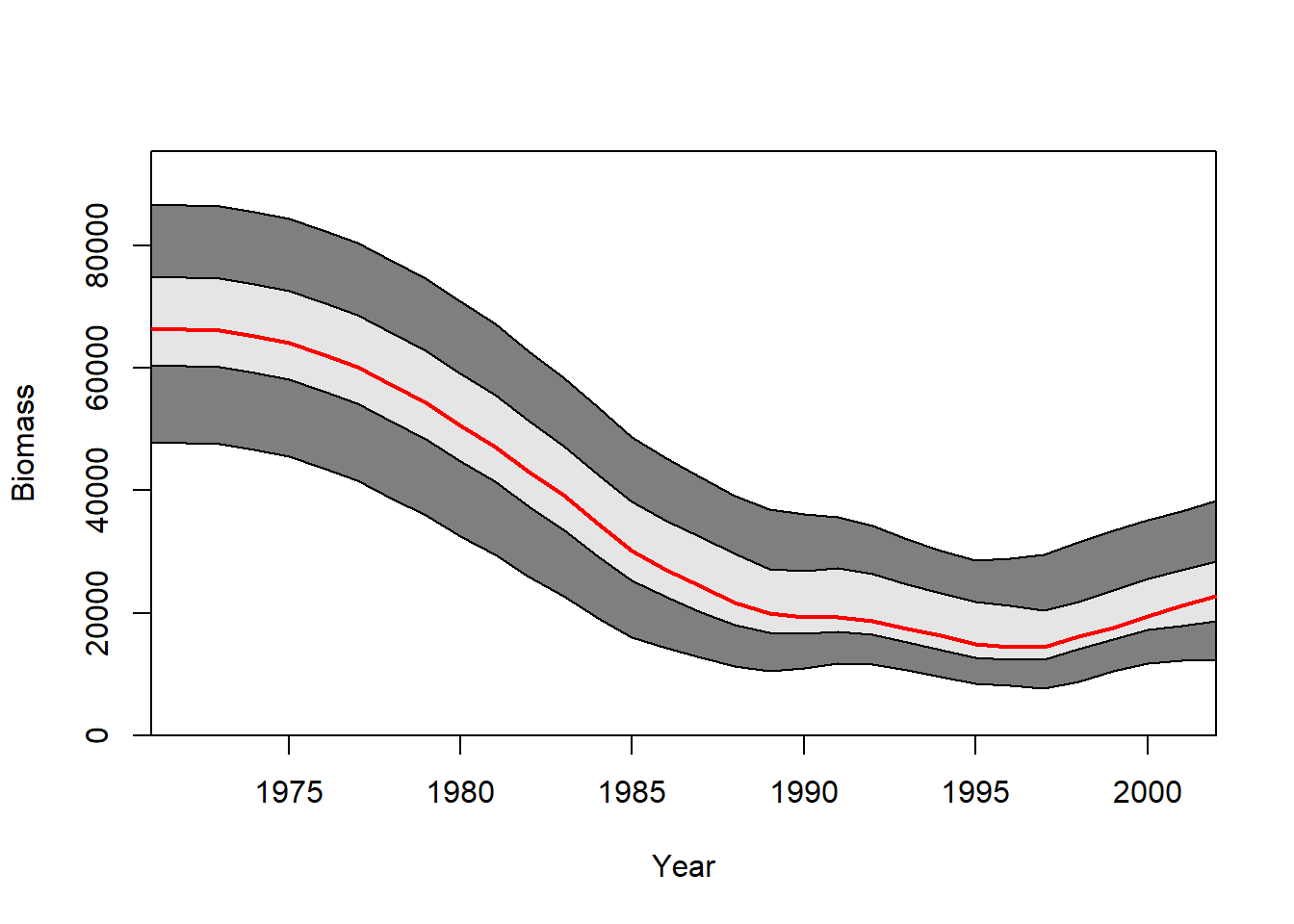## Interpreting results in a management context

Stock status (biomass relative to carrying capacity) can be evaluated from the Bt/K output using dbsraOut\$Parameters. It is possible to conduct projections based on the results of DB-SRA using the dlproj function. The key inputs for this function are:

• dlobj: the output object from dbsra.
• projyears: the number of projection years
• projtype: 0 - median MSY; 1 - mean MSY; 2 - user-specified catch
• projcatch: the vector of projected catches
``ProjRes <- dlproj(dlobj=dbsraOut,projyears=5,projtype=2,projcatch=c(1400,1400,1000,1000,100))``